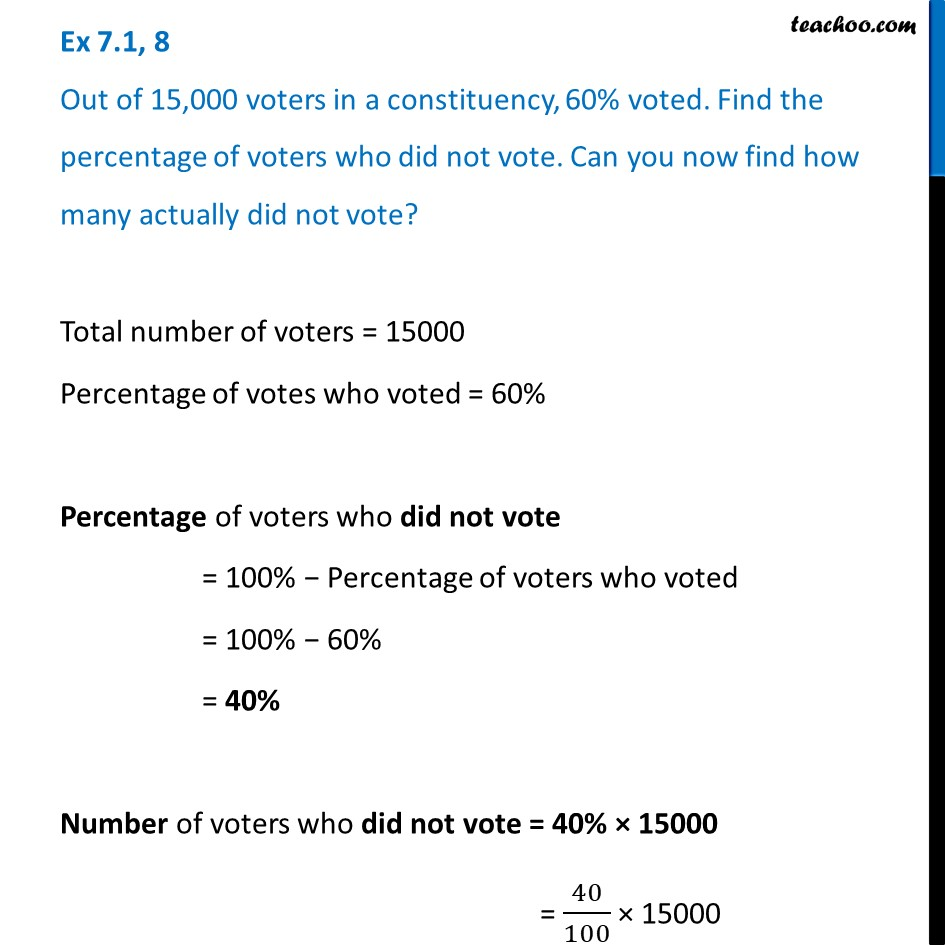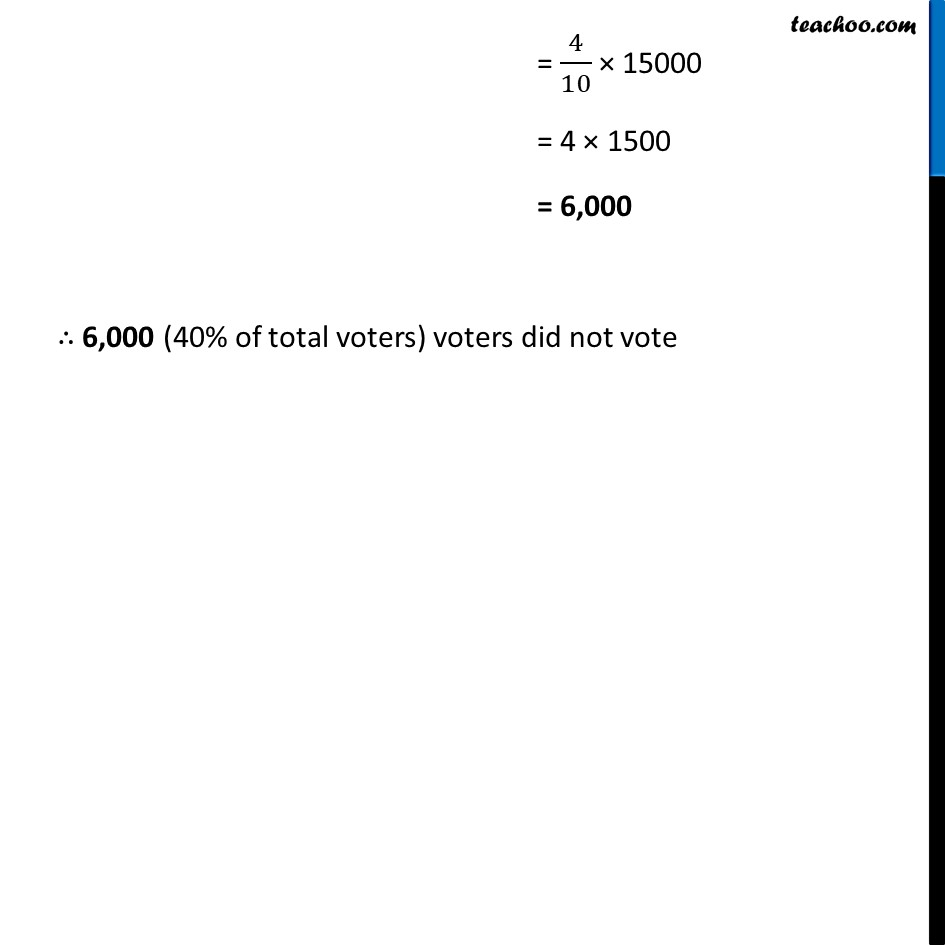Ex 7.1

Chapter 7 Class 7 Comparing Quantities
Serial order wiseLearn in your speed, with individual attention - Teachoo Maths 1-on-1 Class

### Transcript

Ex 7.1, 8 Out of 15,000 voters in a constituency, 60% voted. Find the percentage of voters who did not vote. Can you now find how many actually did not vote? Total number of voters = 15000 Percentage of votes who voted = 60% Percentage of voters who did not vote = 100% − Percentage of voters who voted = 100% − 60% = 40% Number of voters who did not vote = 40% × 15000 = 40/100 × 15000 = 4/10 × 15000 = 4 × 1500 = 6,000 ∴ 6,000 (40% of total voters) voters did not vote